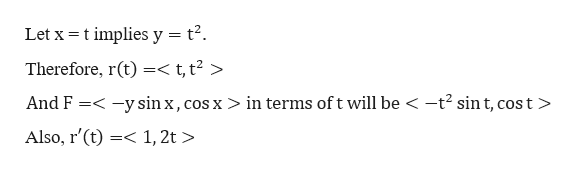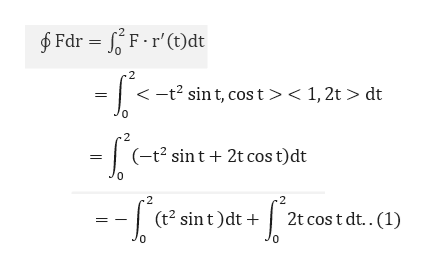# Evaluate ʃC F dr where C is the parabola y = x2 from (0, 0) to (2, 4) and F (x, y) =< −ysinx, cosx >

Question
2 views

Evaluate ʃC F dr where C is the parabola y = x2 from (0, 0) to (2, 4) and F (x, y) =< −ysinx, cosx >

check_circle

Step 1

Given a curve C is a parabola from (0, 0) to (2, 4)

Step 2

We write the curve C in the vector form.help_outlineImage TranscriptioncloseLet x = t implies y = t². Therefore, r(t) =< t, t² > And F =< -y sin x, cos x > in terms of t will be < -t? sin t, cost > Also, r'(t) =< 1, 2t > fullscreen
Step 3

Now, calculate the...help_outlineImage Transcriptionclose\$ Fdr = F.r'(t)dt .2 <-t? sin t, cost>< 1, 2t > dt (-t2 sint + 2t cos t)dt .2 -2 2t cos t dt..(1) (t? sint)dt + fullscreen

### Want to see the full answer?

See Solution

#### Want to see this answer and more?

Solutions are written by subject experts who are available 24/7. Questions are typically answered within 1 hour.*

See Solution
*Response times may vary by subject and question.
Tagged in

### Other